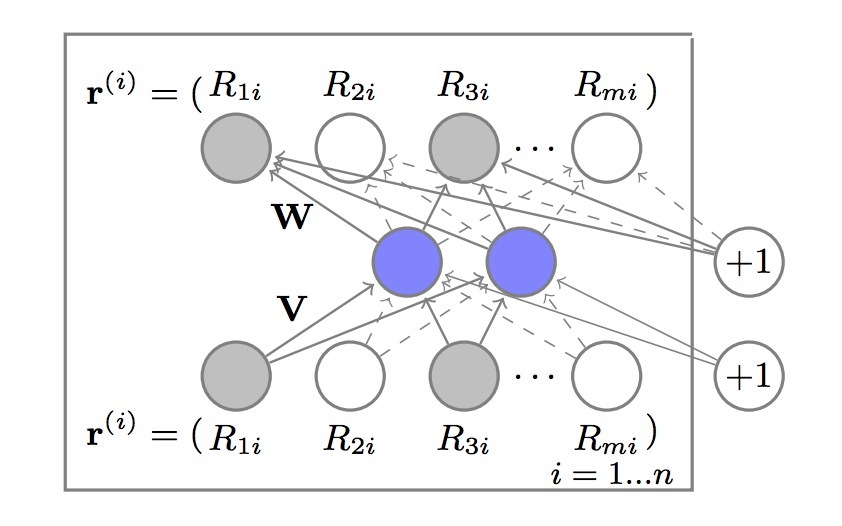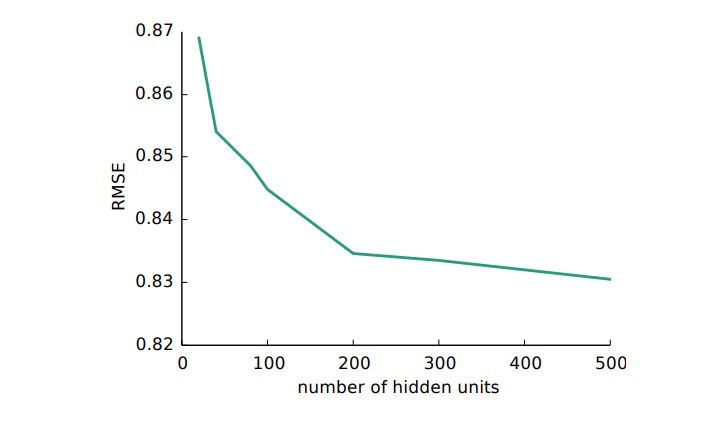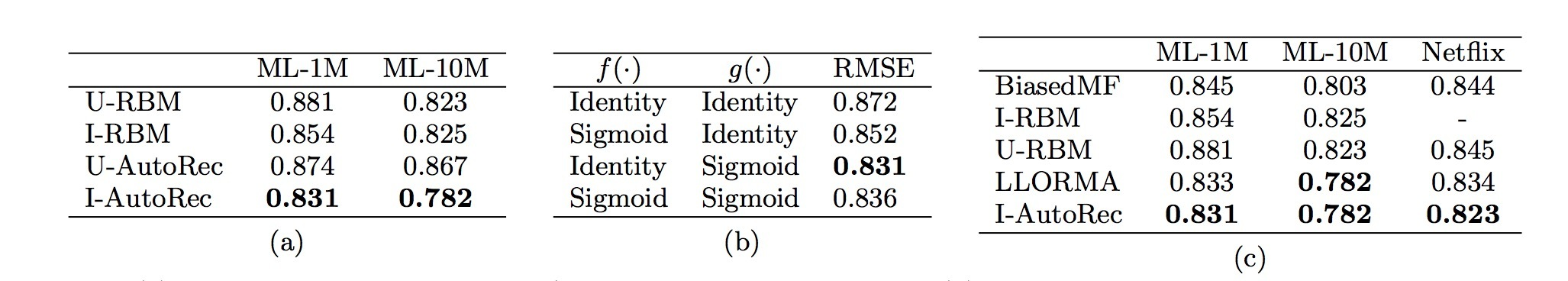1.介绍

CF模型的目的是，根据用户对items（评分）的偏好进行探索，从而来提供个性化推荐。Netflix竞赛提出了一全套不同的CF模型，比较流行的方法有：矩阵分解[1,2]以及邻近模型。该paper提出了AutoRec，一种新的基于autoencoder范式的CF模型。它的灵感原自于最近在视觉和语音任务上的深度学习上获得的成功。AutoRec对于已经存在的CF上的神经网络方法在表征和计算上均有优势，我们会展示它的效果要好于state-of-art方法。

2.AutoRec模型

• 一个部分可观察（相对的，有一部分missing）到的user-item评分矩阵$R \in R^{m \times n}$
• 每个用户$u \in U = \lbrace 1, ..., m \rbrace$，可以被表示成一个部分可观察向量（partially observed vector）：$r^{(u)} = (R_{u1}, ..., R_{un}) \in R^n$

• $r^{(i)}=(R_{1i}, ..., R_{mi}) \in R^m$

$min_{\theta} \sum_{r \in S} \| r - h(r;\theta) \|_2^2$

…(1)

$h(r;\theta) = f(W \cdot g(Vr + \mu) +b)$item-based AutoRec模型，如图1所示，使用一个autoencoder作为等式(1)到向量集合${r^{(i)}}_{i=1}^n$中，有两个重要变化。第一，我们会解释：每个$r^{(i)}$通过在BP期间的更新上关权重来被部分观测，这一点与矩阵分解和RBM方法相同。第二，我们会对学习参数进行正则化，以便防止在观测到的ratings上overfitting。正式的，Item-based AutoRec (I-AutoRec)模型的目标函数是：

$min_{\theta} \sum_{i=1}^{n} \| r^{(i)} - h(r^{(i)};\theta)) \| _O^2 + \frac{\lambda}{2} \cdot ( \| W\|_{F}^2 + \| V \| _{F}^2)$

…(2)

$\hat{R}_{ui} = (h(r^{i}; \hat{\theta}))_u$

…(3)

AutoRec与已经存在的CF方法不同。对比RBM-based CF模型，有一些不同之处：

• 1.RBM-CF提出了一种通用的概率模型，它基于Boltzmann机；而AutoRec是一个判别模型（discriminative model），它基于autoencoders
• 2.RBM-CF通过最大化log似然来估计参数，而AutoRec直接最小化RMSE（在评分预测上的标准评判指标）。
• 3.训练RBM-CF需要使用对比散度（ contrastive divergence），而训练AutoRec需要比较快的基于梯度的BP算法。
• 4.RBM-CF也用于离散评分，并每个评分值估计一个独立的参数集合

3.实验评估

AutoRec的效果随线性和非线性激活函数$f(\cdot)$是如何变化的？表1b展示了在hidden layer中的非线性（通过$g(\cdot)$）对于I-AutoRec上取得好效果是很重要的，它比MF更好。将sigmoid替换为Relu效果会差些。所有AutoRec实验使用标准的$f(\cdot)$和sigmoid $g(\cdot)$函数。

AutoRec的hidden units数目与效果是啥关系？在图2中，我们评估了AutoRec模型的效果，AutoRec会随着hidden units数目变化，并且收益递减。所有AutoRec实验使用k=500.AutoRec的效果与所有baseline相比如何？表1c展示了AutoRec会一直好于所有baseline。参考

http://users.cecs.anu.edu.au/~u5098633/papers/www15.pdf

Updated on

STAR算法介绍

PLE介绍

Published on March 04, 2021

DTS介绍

Published on January 02, 2021